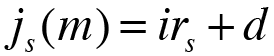The principal   C0 Number of years   n Number of shorter time units in one year   m Annual payment (1) Semiannual payments (2) Four-monthly payments (3) Quarterly payments (4) Bimonthly payments (6) Monthly payments (12) Weekly payments (52) Daily payments (365) Other: Margin or spread   d

Enter the corresponding rate of interest of each year

 Year 1 i1 =Annual effective rate of interestEffective rate of interest per time unitNominal rate of interest
Calculate more precisely

In order to obtain the periodic amortization payments ( as ) of any loan using the adjustable fixed-rate loan method, you have to cancel off the loan at the beginning of each interest adjustments periods theoretically. Then, you have to take out a "new" loan with the corresponding outstanding balance of the previous one ( Cs-1 ).

Each one of these "new" loans are calculated with the rate of interest of each interest adjustments periods and with the remaining term to maturity as the term of the new loan.

You have to know that the index rate ( irs ) of each year has to be adjusted by the margin or spread ( d ), with the exception of the first year interest rate, which is not subject to this type of adjustment. The adjustment is calculated on the basis of the following formula:With the above formula you can obtain the nominal rate of interest of this period ( j(m) ) and you need the effective rate of interest per time unit ( i(m) ), this is why you have to do the following conversion:Once calculated the rate of interest applied to each period, you have to calculate the outstanding debt at the beginning of the period ( Cs-1 ) and the periodic amortization payments of the perido ( as )

The periodic amortization payments ( as ) are calculated on the basis of the following formula:In the first year, you do not need to calculate the outstanding debt ( Cs-1 ), since it is the principal ( C0 ). But, you have to know the outstanding debt ( Cs-1 ) in the remaining year in order to apply the previous formula. So, you have to obtain the outstanding debt ( Cs-1 ) at the beginning of each period on the basis of the following formula:To obtain all the periodic amortization payments ( as ) of the loan, you have to apply these formulas until you have calculated the periodic amortization payments of the last year ( an ).

You should know this: